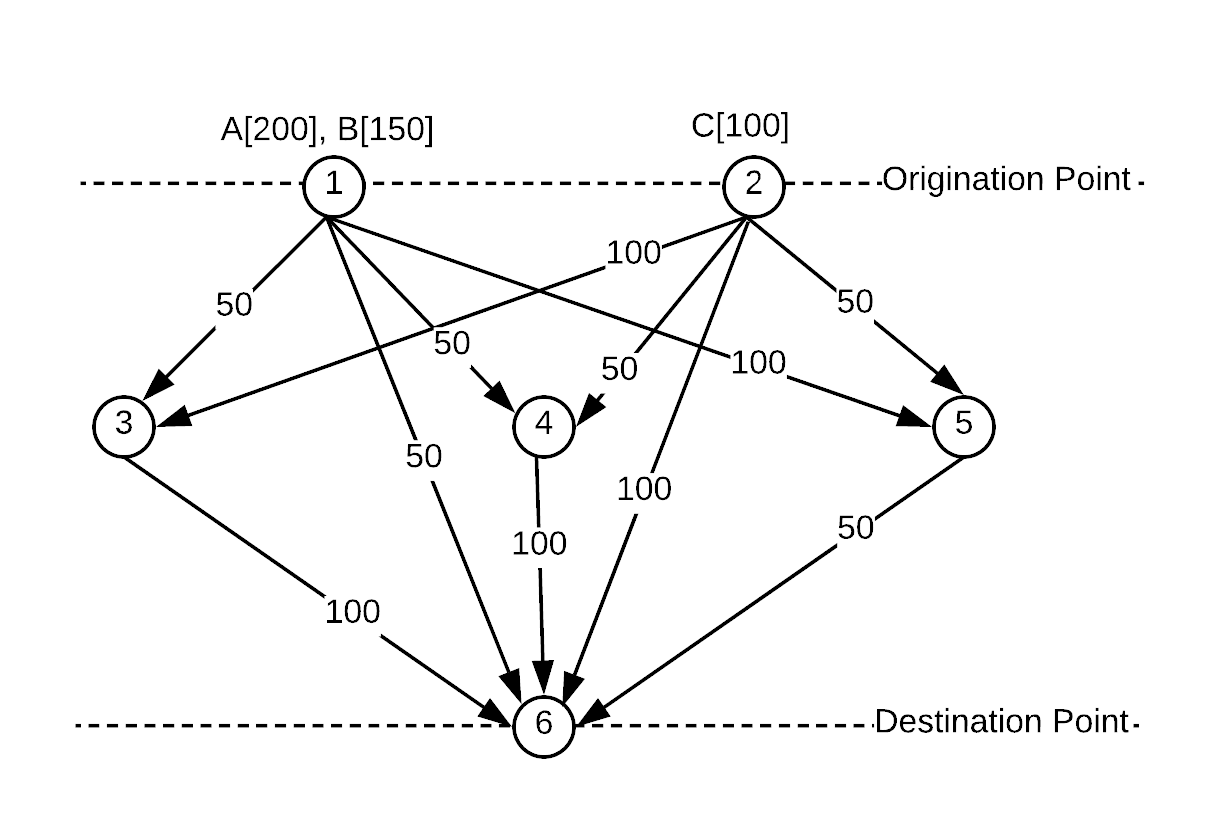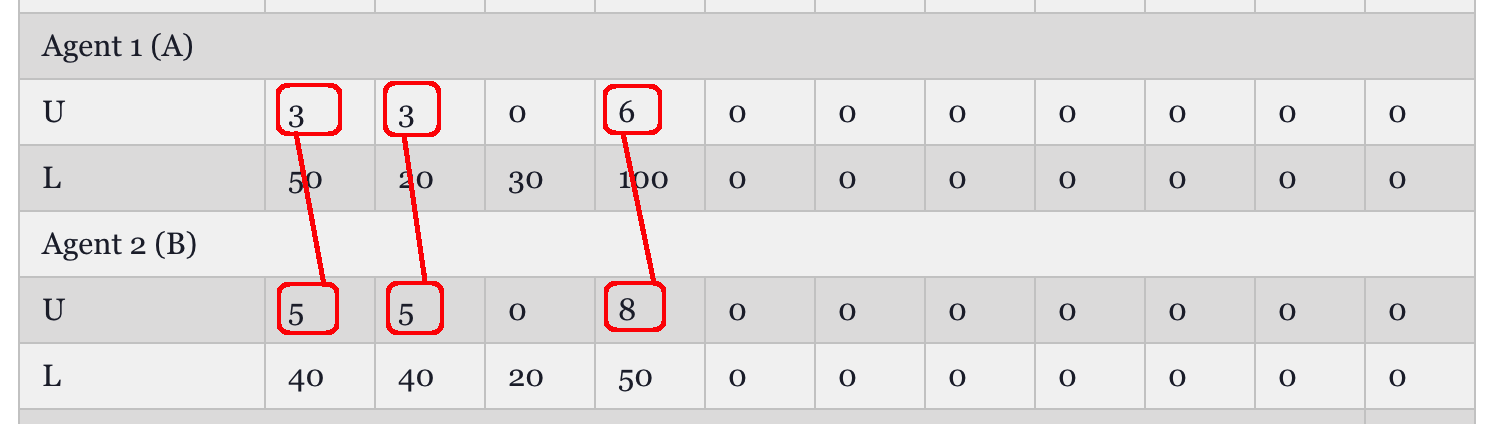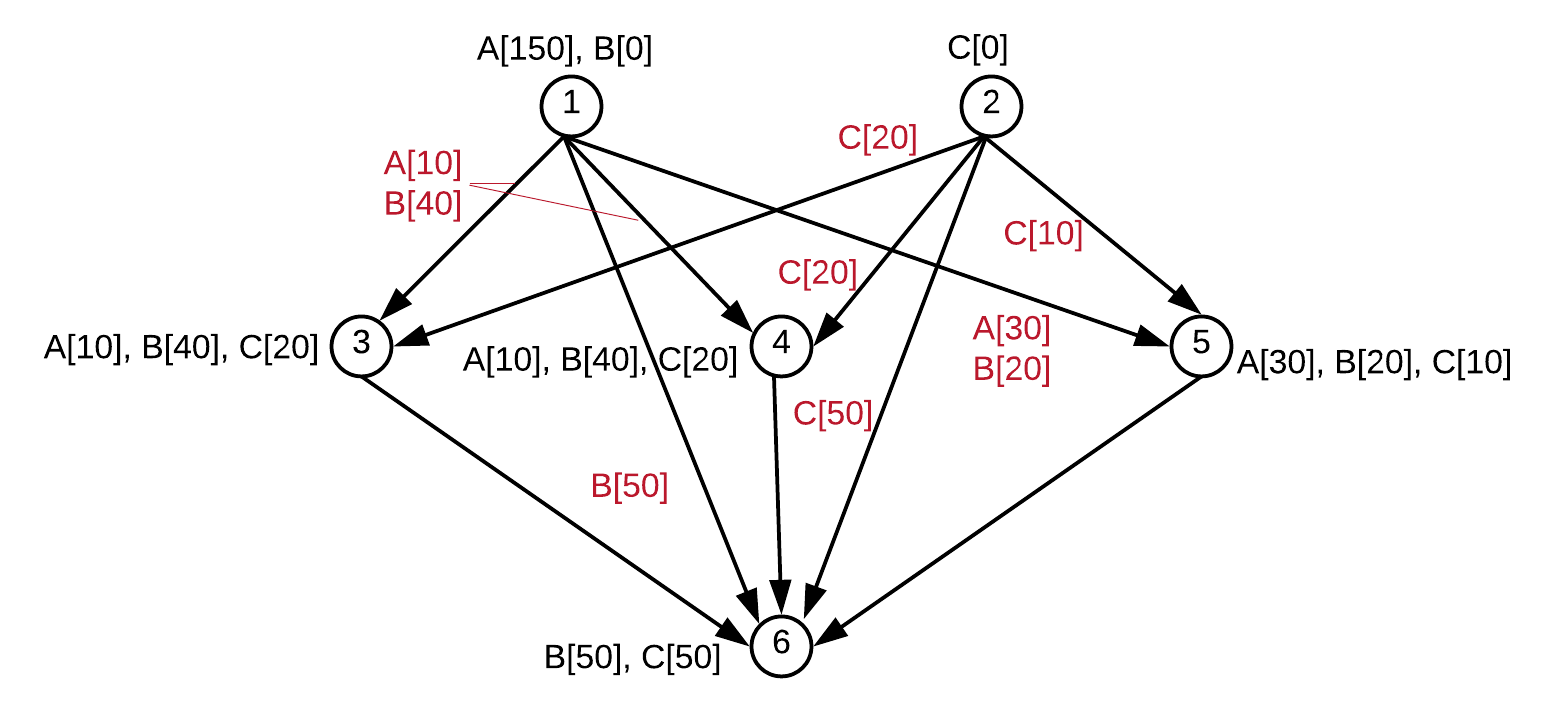{{announcement.body}}
{{announcement.title}}

# Game Theory With Apache Spark, Part 1

DZone 's Guide to

# Game Theory With Apache Spark, Part 1

### In the first of this four-part series, we introduce the topic of game theory, some algorithms from game theory, and how it applies to software.

· Big Data Zone ·
Free Resource

Comment (0)

Save
{{ articles.views | formatCount}} Views

## Introduction

In general terms, Game Theory is concerned with decision making in an environment that involves multiple entities with possibly conflicting interests (Myerson, Roger B. (1997). Game Theory: Analysis of Conflict, Harvard University Press, Wikipedia). Game theory is particularly useful in Prescriptive Analytics where techniques from game theory can be employed to find optimal solutions to complicated decision making problems (for example, see this discussion).

In this article, we will formulate a general resource allocation problem that can be solved using a particular game theory algorithm based on economist Ausubel's Efficient Dynamic Auction Method. We will then implement the algorithm in Apache Spark Machine Learning Library using Java and demonstrate how it works via examples.

This article is organized as follows. In the following section, 'Resource Allocation Via Game Theory' we present various examples from recent literature that discuss using game theory for resource allocation. Then we will introduce the specific resource allocation problems discussed in this article and explain it via two examples. One of the examples involves allocating cloud computing resources to computing clients. The other example is concerned with transportation of various types of goods using shared transportation resources. The following section, 'Algorithm,' discusses an algorithm that achieves an optimal solution for the specific resource allocation problem introduced earlier. For quick reference, that section also provides a table that summarizes all the symbols and definitions discussed so far. In 'Code Review,' we will discuss how to implement the algorithm using Apache Spark Machine Learning Library (the code discussed in that section can also be downloaded from GitHub). The final section 'Conclusions' will give some concluding remarks.

## Resource Allocation Via Game Theory

One application of game theory is finding optimal resource allocation. For example, as discussed in this article, resource management for heterogeneous wireless networks involves sharing network links, e.g. 3G, Wi-Fi, WiMAX, LTE, between mobile devices of different types and different bandwidth needs. In such environments, game theory algorithms can be effectively used to decide which devices must be allocated to which network resources. Similarly, game theory can be used for allocation of cloud computing resources, e.g. CPU, storage, memory or network bandwidth, between resource clients, as discussed in this article (also see here). The concept of Mobile Edge Computing, where mobile devices offload computationally intensive tasks to the small scale computing servers located in the network edge, could utilize game theory concepts for resource allocation, as studied here

Using game theory for resource allocation is not limited to cloud computing or telecommunications. For example, in a recent study, a technique was developed based on game theory for efficient distribution of water supply to consumers. Optimum decision making for traffic flow control at major traffic intersections can also be modeled using concepts from game theory, as studied in this article.

The particular resource allocation problem we consider has the following elements.

• Resource: An item that should be shared between multiple entities. There could be multiple resource types. Availability of a resource type is measured in integer units. Each resource type has a price per unit. There are K > 0 resource types.

• Agent: An entity that needs resources. There are N > 0 agents. An agent may need different types of resources, at different quantities and agent's consumption set indicates at most how many units of a particular resource the agent needs. Each agent has a value function that represents the value the agent obtains from the resources allocated to it. Each agent also has a utility function that combines value and cost due to price of resources.

• Resource Manager: A single entity that makes the decision how to allocate resources to agents. Resource manager sets the price for each resource.

Note that Commodity, Bidder and Auctioneer are equivalent terms to Resource, Agent, and Resource Manager, respectively, in the literature for dynamic auctions with game theory.

### Example 1: Allocation of Cloud Computing Resources

A cloud computing provider has nine resource types (K = 9) to allocate. The resource types represent various kinds of CPU, memory or network links as shown in the following table.

 Resource Type 1 2 3 4 5 6 7 8 9 Description Single-Core CPU 2-Core CPU 4-Core CPU 8-Core CPU 2G RAM 4G RAM 8G RAM 500KB/s Network Link 1MB/s Network Link Available Units 4 2 1 1 4 4 1 4 2

Table 1

For resource type j let Sdenote the number of available supply units, j = 1, ..., K. Then, a vector S is defined by:

S := [S1  S2  ... SK]T

with T representing matrix transpose. There are four agents to allocate those computing resources, i.e. N = 4. Each agent has defined an integer number ≥ 0 for each resource type to indicate how important that resource type is for its needs; for agent i and resource type j let uij ≥ 0 denote that number.

The numbers uij represent the value of resource types for the agents and therefore we call uij the value coefficients, i = 1, ..., N, j = 1, ..., K. Note that the larger the uij, the more important that resource type is for the particular agent. Also each agent has designated an upper bound to each resource type to indicate at most how many of units of that resource type it needs; for agent i and resource type j let Lij ≥ 0 denote that number. If an agent does not need any units of a particular resource type, i.e. Lij = 0 then uij = 0. In general, Lij and uij are defined before the resource manager sets the price for each resource type.

The below table gives uij and Lij, i = 1,...,4, j = 1,...,9: for agent i the value of row U under the column for Resource Type j is uij and the value of row L under the column for Resource Type j is Lij.

 Resource Type 1 2 3 4 5 6 7 8 9 Description Single-Core CPU 2-Core CPU 4-Core CPU 8-Core CPU 2G RAM 4G RAM 8G RAM 500KB/s Network Link 1MB/s Network Link Agent 1 U 0 2 3 5 2 3 6 3 6 L 0 8 4 2 8 4 2 8 4 Agent 2 U 2 3 0 0 2 4 0 3 5 L 4 4 0 0 8 6 0 4 2 Agent 3 U 1 2 3 3 1 2 3 1 1 L 1 2 4 2 4 2 1 8 4 Agent 4 U 5 2 0 0 3 2 0 4 0 L 2 1 0 0 2 1 0 1 0

Table 2

The resource manager determines prices for each resource type and the corresponding allocations, which are 'optimal' (Ww will discuss the concept of optimality shortly).

The below table shows the optimal price and allocations. Under a resource type column the number indicates the corresponding price across the Price row. Similarly, under a resource type column the number indicates how many units of that type is allocated across the row for each particular agent (rows Agent 1 - Agent 4).

 Resource Type 1 2 3 4 5 6 7 8 9 Description Single-Core CPU 2-Core CPU 4-Core CPU 8-Core CPU 2G RAM 4G RAM 8G RAM 500KB/s Network Link 1MB/s Network Link Price 2 3 3 5 2 4 6 3 6 Agent 1 0 0 1 1 2 0 1 3 2 Agent 2 2 2 0 0 0 4 0 0 0 Agent 3 0 0 0 0 0 0 0 0 0 Agent 4 2 0 0 0 2 0 0 1 0

Table 3

As expected, allocated units of resource type j to agent i does not exceed Lij.  In addition, for resource type j, sum of allocated units to all agents equals available units Sj, j = 1,...,9 (see Table 1).

## Price and Optimality

We will now discuss how the prices and allocations above are optimal. For that purpose, we have to introduce some definitions.

Let P := [p1 p2 ... pK]T be the price vector.

For agent i let Xi be the set of all K-dimensional nonnegative integer vectors the agent could accept as part of an allocation. For a vector in Xi, dimension j corresponds to a particular resource type and is less than or equal to Lij. For example, for agent 1, consider the L row from Table 2:

• [0    8    4    2    8    4    2    8    4]

Notice the three examples below are elements of X1:

• [0 8 4 2 8 4 2 8 4]T

• [0 7 4 2 8 4 2 8 4]T

• [0 8 3 2 8 4 2 8 4]T

However, [1 8 4 2 8 4 2 8 4]T cannot be an element of X1 because its 1-st entry, 1, is greater than zero and thus exceeds L11 (= 0).

For agent i = 1,...,N, Xi is defined as the consumption set.

Next, for i = 1,...,N define a K-dimensional integer vector:

Ui := [ui1 ui2 ... uiK]T

where uij ≥ 0 are called the value coefficients.

For allocation x := [xi1 xi2 ... xiK]T ∈ Xi to agent i, we define the value function as follows:

Ui(x) := UiT  * x

where * denotes matrix multiplication. The function Ui(x) - P*x is called the utility of agent i at price P. Utility is the value agent gets from the resources allocated to it minus how much it pays for them.

Define

Vi(P) := max x ∈ X{Ui(x) - P * x}

Vi(P) is defined as the maximum utility agent i gets at price P where the maximum is defined over the agent's consumption set.

Define Qi(P) to be a subset of Xi where each element of Qi(P) achieves the maximum utility at that price. In mathematical terms,

Qi(P) := arg max x ∈ X{Ui(x) - P * x}

Notice that we introduce Qi(P) as a set, implying that more than one element in an agent's consumption set could achieve the maximum utility at price P.

Then, an optimal price Po and a corresponding allocation xoij of resource types j = 1,...,K to agents i = 1,...,N satisfy the following:

Condition 1: For all resource types all available units are allocated:

i ∈ {1, 2, ..., N}  xoij = Sj, ∀ j ∈ {1,...,K}

Condition 2: For each agent the number of allocated units of a resource type cannot exceed the upper bound the agent has designated for that resource type (equivalently, the allocated units belong to the consumption set of the agents):

Lij ≥ xoij, ∀ i ∈ {1,...,N}, ∀ j ∈ {1,...,K}

Condition 3: Any allocation [xoi1  xoi2  ...  xoiK]T corresponding to an optimal price Po achieves the maximum utility at Po for every agent:

[xoi1 xoi2  ... xoiK]T∈ Qi(Po), ∀ i ∈ {1,...,N}

Condition 4: Po is the highest price for which conditions 1 - 3 above hold. That is, for any P where conditions 1 - 3 are satisfied, Po ≥ P. (By definition, if vectors a, b satisfy ab then every element of a is greater than or equal to the corresponding element of b.)

• Notice that there is a fairness-based equilibrium introduced by the above conditions: while every agent maximizes its utility the resource manager maximizes the price of the resources it is allocating. In addition, while all the available units are allocated across all resource types no agent is forced to take more than it needs, although it could get less.

• To satisfy condition 1, for each resource type, sum of the upper bounds the agents have designated for that resource type should not fall short of the available units for the resource type. To guarantee condition 1 holds, an auxiliary agent may need to be introduced. The auxiliary agent bids for any outstanding supply units not needed by actual agents and issues 0 value for each resource type, to avoid competition (see example 2 below).

• For the theoretically inclined reader, the conditions 1 - 4 above constitute a Walrasian (a.k.a.  competitive) equilibrium.

The above concepts suggest an environment where entities act in self-interest competing against each other. However, those concepts can also be applied to situations where a single unit, e.g. a corporation, allocates its resources optimally within the entities it owns. The second example below will highlight that scenario.

### Example 2 - Transportation of Goods

Consider three types of goods, A, B ,and C to be transported from an origination point to a destination point. There are 200, 150, and 100 units of goods A, B and C, respectively. At each execution step, certain number of goods will be transported from one node to another as a batch. Transportation links between the nodes have certain capacities indicating how many total units of any type of good can be carried over them at a given step. For example, between nodes 1 and 3 a total of 50 units of goods can be carried at a given step. The origination point for type A and B goods is node 1 and the origination point for type C goods is node 2. The destination point for all goods is node 6. The carrier would like to prioritize shipping of type B goods over others. The carrier formulates a resource allocation problem where each transportation link is a resource type with certain number of available units (link capacity). For each agent, A, B and C, and for each transportation link a value is set representing value of the particular transportation link for the agent. The values are higher for agent B than agents A, C because shipping type B goods has priority.

The below figure depicts the above scenario where circles indicate nodes and directed lines indicate transportation links. A number inside a circle is node's identifier and a number over a line is link's capacity. Numbers inside brackets A[.], B[.], C[.] show the number of units for the corresponding resource type at the particular node in current state.Figure 1. Initial setup for transportation of goods.

For step 1, the formulation is as follows. The row S defines link capacities. For an agent, the corresponding row U defines the value of each transportation link; the row L indicates at most how many units of that transportation link the agent needs. Also, an auxiliary agent 4 has been introduced to bid against unused capacity in transportation links.

Table 4

Because type B has been prioritized higher than type A, the corresponding value function has larger value coefficients, e.g. 8 vs. 6 or 5 vs. 3:Figure 2. Value coefficients.

For step 1, the algorithm yields the following optimal allocation.

Table 5

At end of step 1, goods are transported based on the above allocation to achieve the state summarized in below figure, where number of goods transported over each link is highlighted in red.Figure 3. Transportation of goods after step 1 is completed.

After introducing the algorithm and the corresponding code review, we will explain how to obtain the above allocation and continue with the example to complete all the steps in 'Bringing Pieces Together - Example 2' below.

That's all for Part 1! Tune in tomorrow when we'll discusss some interesting algorithms.

Topics:
apache spark, artifical intelligence, big data, game theory

Comment (0)

Save
{{ articles.views | formatCount}} Views

Opinions expressed by DZone contributors are their own.